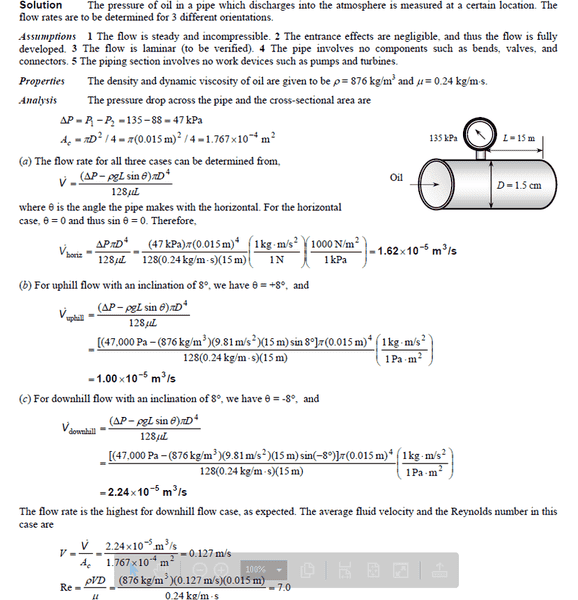# Pressure in inclined pipe

## Homework Statement

in the solution , the author gave that volume flow rate = delta P - (rho)(g)(L sin theta) ,
IMO , it's wrong ...## The Attempt at a Solution

it should be P1 - (rho)(g)(L sin theta) , where (rho)(g)(L) = P2 , correct me if i am wrong . i think so because i consider P1 - (rho)(g)(L sin theta) as delta P

Chestermiller
Mentor
P2 is zero gauge. the pipe discharges to the atmosphere.

•foo9008
P2 is zero gauge. the pipe discharges to the atmosphere.
why the author wrote it as delta P - (rho)(g)(L sin theta) ? why not only delta P , which is Pressure at region 1 only ( the P2 = 0 ) ??? why there's an extra (rho)(g)(L sin theta) ?

Chestermiller
Mentor
why the author wrote it as delta P - (rho)(g)(L sin theta) ? why not only delta P , which is Pressure at region 1 only ( the P2 = 0 ) ??? why there's an extra (rho)(g)(L sin theta) ?
Are you familiar with the Navier Stokes equations?

•foo9008
Are you familiar with the Navier Stokes equations?
no , i have never heard of that

Chestermiller
Mentor
If the fluid were in static equilibrium, then we would have $$P_1+\rho g z_1=P_2+\rho g z_2$$In this problem, they are taking the datum for elevation z as location 1, the entrance to the pipe section. So ##z_1=0##. So for static equilibrium, our equation becomes:$$P_1=P_2+\rho g z_2$$ The elevation ##z_2## is related to the length of the pipe and the pipe angle by:$$z_2=L\sin \theta$$. Therefore, for static equilibrium, we have:
$$P_1=P_2+\rho g L\sin \theta$$Now, if ##P_1>P_2+\rho g L\sin \theta##, the fluid will flow from point 1 (the inlet) to point 2 (the outlet). Therefore, the driving force for fluid flow from entrance to exit is ##P_1-P_2-\rho g L\sin \theta=\Delta P-\rho g L\sin \theta##.

•foo9008